Geosci. Instrum. Method. Data Syst., 10, 153–160, 2021
https://doi.org/10.5194/gi-10-153-2021
Geosci. Instrum. Method. Data Syst., 10, 153–160, 2021
https://doi.org/10.5194/gi-10-153-2021

Research article 23 Jul 2021

Research article | 23 Jul 2021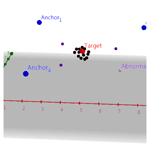# Research on real-time elimination of ultra-wideband radar ranging abnormal value data

Research on real-time elimination of ultra-wideband radar ranging abnormal value data
Xin Yan, Hui Liu, Guoxuan Xin, Hanbo Huang, Yuxi Jiang, and Ziye Guo Xin Yan et al.
• School of Electrical and Information Engineering, Beijing University of Civil Engineering and Architecture, Beijing 100044, China

Correspondence: Hui Liu (liuhui@bucea.edu.cn)

Abstract

For indoor positioning, ultra-wideband (UWB) radar comes to the forefront due to its strong penetration, anti-jamming, and high-precision ranging abilities. However, due to the complex indoor environment and disorder of obstacles, the problems of diffraction, penetration, and ranging instability caused by UWB radar signals also emerge, which make it difficult to predict the noise and leads to a great impact on the accuracy and stability of the measurement data in the short term. Therefore, the abnormal value migration of the positioning trajectory occurred in real-time positioning. To eliminate this phenomenon and provide more accurate results, the abnormal values need to be removed. It is not difficult to eliminate abnormal values accurately based on a large number of data, but it is still a difficult problem to ensure the stability of the positioning system by using a small number of measurement data in a short time to eliminate abnormal value in real-time ranging data. Thus, this paper focuses on the experimental analysis of a UWB-based indoor positioning system. By repeatedly measuring the range , a large number of measurement data can be obtained. Using the massive data to train linear regression models, we get the parameter of the linear model of range data measured with the UWB radar. Based on the Gaussian function outlier detection, abnormal values are eliminated, and putting the new range data into the regression model trained by us, the ranging error is reduced by nearly 50 % compared with the peak and mean ranging errors in general.

Share
1 Introduction

Ultra-wideband (UWB) technology, due to its high transmission rate, penetration, security, and low system complexity, has been favored by many scholars in the field of indoor positioning. In 2014, Khajenasiri et al. (2014) developed a low-power UWB transceiver for smart home energy consumption monitoring and management, which is 1 order lower than the commercial wireless technology applied in smart home applications. In 2017, Mokhtari et al. (2017) put forward the use of UWB technology to monitor some high-risk areas in a smart home environment. In 2012, Madany et al. (2012) investigated ITS and proposed the use of UWB technology in vehicle-to-vehicle and vehicle-to-infrastructure communication of multi-user ITS technology. In 2018, Mostajeran et al. (2018) proposed a UWB full-scale imaging radar with Asia–Pacific hertz frequency. This was the first terahertz/sub-terahertz frequency imaging radar providing good lateral resolution without any focal lens or reflector. For objects with a distance of 23 cm, it achieved 2 mm lateral resolution and 2.7 mm range resolution.

In 2016, Kim and Choi (2016) proposed an automatic landing system for an unmanned aircraft system (UAS) based on the UWB, optimized the geometric structure of the UWB anchor in the network, and achieved a more accurate positioning performance for the UAS landing process. In 2018, Nakamura et al. (2018) studied a pedestrian positioning system based on UWB ranging. In this system, the base station receiving the UWB signals transmitted by the pedestrians was connected to the traffic lights, and the locations of pedestrians were estimated by the least squares method using the distance estimated by the UWB ranging scheme. In 2017, Kolakowski (2017) proposed the concept combining Bluetooth low-power (BLE) and UWB positioning to improve the energy efficiency. In 2017, Ruiz et al. (2017) compared the performance of three commercial UWB systems, namely Ubisense, BeSpoon, and DecaWave, under the same experimental conditions. A measurement model combining Bayesian and particle filters was used. The model considered errors in distance measurement and found the abnormal values. The results indicated which system performed better under these industrial conditions. In 2015, Ledergerber et al. (2015) proposed a self-positioning robot system based on one-way UWB communication. By passively receiving the UWB radio signals from a fixed position, the position of the robot in a certain space was estimated. In 2016, Hepp et al. (2016) proposed an omnidirectional tracking system for flying robots based on blocking robust UWB signals. Compared to the typical UWB positioning systems with a fixed UWB converter in the environment, this system only needed one UWB converter to detect the target. In 2017, Perez-Grau et al. (2017) proposed a multi-modal mapping system based on UWB and RGB-D. By using the synergy between the UWB sensor and point cloud, a multi-mode three-dimensional (3D) map with a UWB sensor was generated for location estimation, which was integrated into the Monte Carlo localization method. In 2018, Schroeer (2018) used a real-time UWB multi-channel indoor positioning system for industrial scenes to evaluate multi-path and non-line-of-sight situations. In the same year, Stampa et al. (2018) proposed a semi-automatic calibration method for the UWB-based distance measurement of the autonomous mobile robots. Aiming at the system ranging error observed in the UWB distance measurement, a semi-automatic calibration method was proposed to estimate the error model approximating its influence.

The research on UWB in China, however, started relatively late. Although it is not as mature as that of foreign countries, some works have been done with the strong support of the state. In 2010, Chen et al. (2010) designed a UWB transmitter combined with a digital pulse generator and a modulator to minimize the power consumption. In 2013, Wang et al. (2013) proposed the use of UWB technology to monitor the load in football training. In 2016, Zhang et al. (2016) used UWB radar to image two targets behind the double-wall using the time-domain back projection (BP) and the frequency domain phase shift (PSM) algorithms. In 2017, Ke et al. (2017) proposed an integrated method of intelligent vehicle navigation and positioning based on GPS and UWB. When a vehicle was in a position where the GPS signals were difficult to receive, such as tunnels, the positioning was fulfilled by UWB, and the lost GPS signals were used to replace the integrated positioning of the vehicles. In 2016, Dai et al. (2016) analyzed the main factors affecting the UWB positioning accuracy in a hazardous chemical warehouse and accordingly proposed a UWB four-reference vector compensation method for the stacking location, which was suitable for monitoring the five-segment distance. In 2017, Fu et al. (2017) proposed a method to detect the attitude of the road header by using the UWB ranging technology to realize the unmanned driving.

UWB radar is favored by researchers of indoor positioning systems because of its strong penetration, anti-jamming, and high-precision ranging ability. However, due to the complex indoor environment and the disorder of obstacles, the problems of diffraction, penetration, and ranging instability of UWB radar signals also emerge. Thus, this paper focuses on the experimental analysis of a UWB-based indoor positioning system to further improve the stability and accuracy of UWB radar ranging data. Aiming at the real-time measurement of a large number of UWB radar ranging data, this paper proposes that the processing of the acquired data has to be performed immediately to meet the real-time requirement of the positioning systems. Thus, abnormal values and redundant data in ranging can be removed in real-time, and more accurate and stable results can be delivered to an indoor positioning module.

Since the range information measured in a short time is very unstable and even has abnormal values in the complex indoor environment and unpredictable noise signals, the abnormal values should be eliminated. Based on this, this paper focuses on the experimental analysis of a UWB radar indoor positioning system. To improve the stability of UWB radar ranging data and increase the overall accuracy, this paper studies a large number of UWB radar ranging data by using high-frequency ranging instead of mean value to train estimation model. The high-frequency range value is used instead of the mean value, and the distance estimation model is trained. The abnormal value is detected based on the function, and the abnormal value is removed after training. The ranging error obtained by distance measurement is nearly 50 % lower than that of peak and mean ranging errors.

2 P440 UWB wireless sensor location framework

The P440 UWB wireless sensor operates at a center frequency of 4.3 GHz and has a bandwidth of 2.2 GHz. The signal ranging accuracy of ideal laboratory environment calibration can reach 0.05 m and works well in extremely challenging environments. The test bed for positioning in laboratory using the P440 UWB wireless sensor is shown in Fig. 1.

This experiment is used to realize indoor 3D positioning. Four P440 UWB wireless sensors are used as base stations (also called anchor nodes), and a P440 UWB wireless sensor is used as a node to be tested, which can be installed in mobile devices usually used in indoors (e.g., sports robots). The P440 can obtain the distance information between two nodes. By using this and the positioning algorithm, the t coordinates of each node in 3D can be acquired, and the three-dimensional positioning result of the node to be tested can be obtained.

The experimental results of the 3D positioning in a laboratory environment are shown in Fig. 2. It is found that, due to the complex indoor environment, the interference from indoor objects is relatively serious, resulting in unstable distance information measured in a short period of time, and even abnormal values. Therefore, when performing real-time positioning, the positioning trajectory generates an abnormal value offset phenomenon. In order to improve the stability and accuracy of the real-time positioning, the original ranging data need to be analyzed and processed as reducing the influence of the abnormal values so that the target point to be tested is stabilized in a small range.

3 Ranging data analysis

## 3.1 Distribution function and parameter estimation of the ranging data

In the laboratory environment, two P440 wireless sensors were used to obtain a large number of ranging data from four different distance locations, and Fig. 3 shows the histogram of the data acquired. As seen, the data obtained follow a Gauss distribution, which can be formulated as

$\begin{array}{}\text{(1)}& f\left(x\right)=\frac{\mathrm{1}}{\sqrt{\mathrm{2}\mathit{\pi }}\mathit{\sigma }}\mathrm{exp}\left[-\frac{{\left(x-\mathit{\mu }\right)}^{\mathrm{2}}}{\mathrm{2}{\mathit{\sigma }}^{\mathrm{2}}}\right],\end{array}$

where μ is the mean, and σ2 is the variance.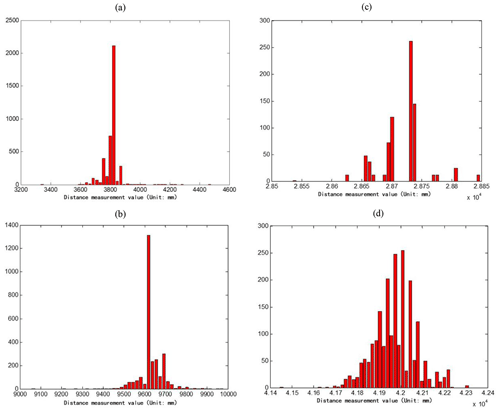Figure 3Histogram of the measured values at different distances. (a) Histogram of the measured value at 3560 mm. (b) Histogram of the measured value at 9640 mm. (c) Histogram of the measured value at 28 220 mm. (d) Histogram of the measured value at 41 540 mm.

The laboratory value fits its probability density distribution curve for these four sets of data, as shown in Fig. 4. As seen, the expected value deviates from the true value: there is a huge difference in the standard deviation of the Gaussian distribution for each group of data. Table 1 gives the expected and standard deviation of the estimated four sets of data. This is an indicator of abnormal values, which causes measurement discrepancy between the measurements and the true values. Thus, the data cannot be used for positioning due to the abnormal values.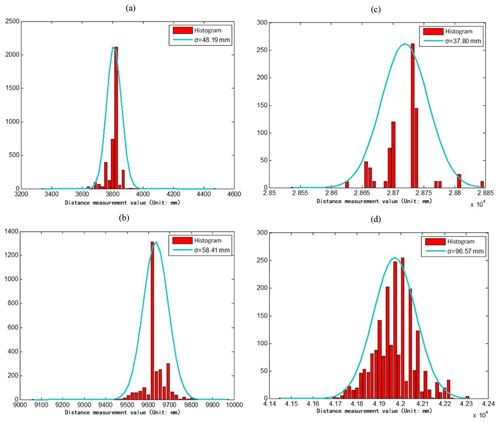Figure 4Fitting results of the probability density distribution curve. (a) Probability density at 3560 mm. (b) Probability density at 9640 mm. (c) Probability density at 28 200 mm. (d) Probability density at 41 540mm.

## 3.2 Mean, peak, and true values of the ranging data

In the above-given measurement data, due to the disturbance of abnormal values, the deviation between the expected, peak, and the true values is high. In positioning, especially for mobile tracking, it is impossible to collect a large number of data in a short time. Therefore, we collected only 60 groups of measurement data from different distances and accordingly calculated their mean and peak values.

## 3.3 Distance estimation model training

Figure 5 and Table 2 show that, using the mean peak as a reference, there exists a determination error between the truth value and the mean peak, but it can be seen that a certain linear relationship will be established between them, as shown in Fig. 6 (scattered dots in red). By using a polynomial, 50 of the 60 sets of data were selected as the training set to fit the linear relationship between the peak and the true values of the measured data, as shown by the solid cyan line in Fig. 6. The other 10 sets are set aside as test sets. Table 2 shows the statistical results of the true, peak, and mean values of the selected 50 groups of data.

Table 2Truth value, (measured) peak value, and (measured) mean value of the 50 training data sets.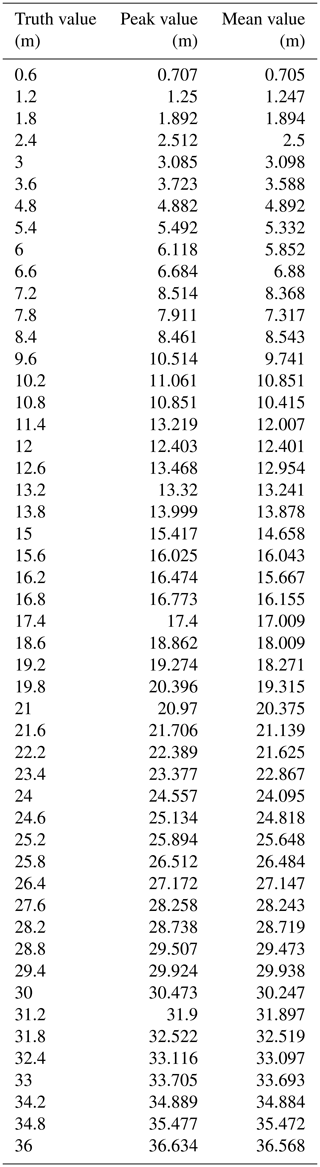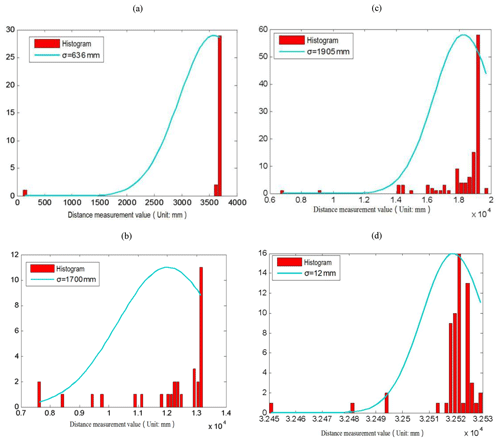Figure 5Statistical and probability density fitting results of measured values with small data volume. (a) Probability density at 3600 mm. (b) Probability density at 11 400 mm. (c) Probability density at 19 200 mm. (d) Probability density at 31 800 mm.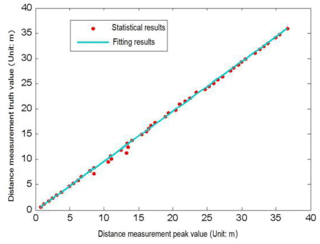Figure 6Statistical results and fitting of the peak and true values of distance measurement.

4 Data processing methods

The statistical results of the peak and true values in Sect. 3 showed that the deviation between the peak and the true values is linear, satisfying a certain relationship. In order to make the measured value as close to the real value as possible, a linear regression is applied to fit the measured value. A large number of measured data are utilized to train the parameter of the linear model. When the optimal model is obtained, the deviation between the measured value and true value could be corrected and reduced as much as possible. Since the repeated and massive measurement data meet the Gaussian distribution, the peak value is taken as the reference mean for processing. Then, the ranging results that the reference mean meets certain conditions are retained, and those that do not meet the conditions: abnormal values are removed. According to the linear model that has been trained by us, the estimated value is more accurate than the measured value. The linear regression results are shown in Fig. 6, and the expression of the linear model can be written as

$\begin{array}{}\text{(2)}& y=\mathrm{0.9859}x-\mathrm{0.1633},\end{array}$

where x is the peak value, and y is the true value.

## 4.1 Gaussian abnormal value detection

Since the distance measurement follows a Gaussian distribution $N\sim \left(\mathit{\mu },{\mathit{\sigma }}^{\mathrm{2}}\right)$, the Gaussian function is used for abnormal value detection. Here, the abnormal values and the normal data are calibrated, the abnormal value data are eliminated, and the normal data are retained.

$\begin{array}{}\text{(3)}& x=\left(y+\mathrm{0.1633}\right)/\mathrm{0.9859}.\end{array}$

Since the measurement data satisfy the Gaussian distribution $N\sim \left(\mathit{\mu },{\mathit{\sigma }}^{\mathrm{2}}\right)$, it is known from Eq. (3) that when the distance estimation is carried out by Eq. (2), the estimated value also satisfies the Gaussian distribution. The estimated mean μy and the standard deviation σy can be expressed as

$\begin{array}{}\text{(4)}& {\mathit{\mu }}_{y}=\mathrm{0.9859}\mathit{\mu }-\mathrm{0.1633},\text{(5)}& {\mathit{\sigma }}_{y}=\mathrm{0.9859}\mathit{\sigma }.\end{array}$

Therefore, if it is desired to obtain an estimated value error less than the threshold δy, the error x between the measured value of δx and the peak value has to satisfy ${\mathit{\delta }}_{x}<{\mathit{\delta }}_{y}/\mathrm{0.9859}.$

According to this method, abnormal values were detected for the four groups of data, and the results are shown in Fig. 7. As seen, the method successfully removes the abnormal values. Among the four groups of data, the first three groups had a very large standard deviation due to the existence of abnormal value. After the elimination of abnormal value, the standard deviation remained within 50 mm.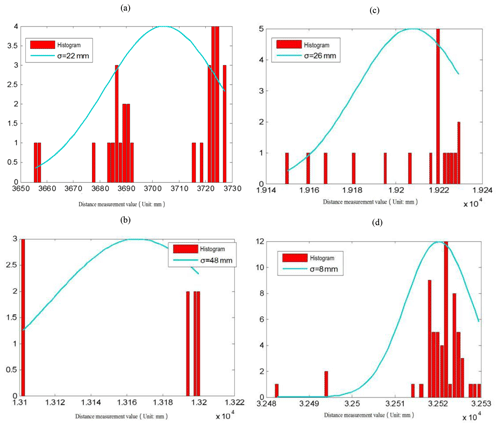Figure 7Statistical results after removing abnormal value. (a) The result of rejecting abnormal value at 3600 mm. (b) The result of rejecting abnormal value at 11 400 mm. (c) The result of rejecting abnormal value at 19 200 mm. (d) The result of rejecting abnormal value at 31 800 mm.

## 4.2 Estimating the truth value

After removing the abnormal value by the Gaussian function, according to the above analysis, the ranging value cannot be directly used for positioning. This is because the error between the ranging peak and the true values of the P440 is still high, and thus the required ranging value needs to be retained. The estimation is performed by using Eq. (2), and the results are shown in Table 3, which gives the estimated values of the 10 sets of test data. Table 4, on the other hand, shows the overall error when estimating the ranging distance by using peaks, expected values, and the methods proposed in this paper.

5 Conclusion

In this paper, the experimental analysis and research on the ranging data of the UWB radar indoor positioning system were carried out. To meet the needs of indoor real-time positioning and further improve the stability of UWB radar ranging data and the overall accuracy, a large number of UWB radar ranging data were studied, and the high-frequency ranging value was used to replace the mean value and train the range estimation model. The abnormal value was detected based on the Gaussian function. After removing the abnormal value, the distance estimation model was used to estimate the distance value. The results showed that the distance measurement error obtained is nearly 50 % lower than the peak and mean distance measurement errors.

Data availability

The data set can be found at https://pan.baidu.com/s/1qCygUi-MeNN-QfOD6K9Cmw (Yan, 2021).

Author contributions

XY and HH designed the experiments, and XY developed the model code. XY, GX, and HH carried them out. XY prepared the manuscript with contributions from all co-authors. HL, YJ, and ZG revised the manuscript.

Competing interests

The authors declare that they have no conflict of interest.

Financial support

This research has been supported by the National Natural Science Foundation of China (grant no. 61501019), the Scientific Research Project of Beijing Educational Committee (grant no. SQKM201710016008), and the Fundamental Research Funds for Beijing University of Civil Engineering and Architecture (grant no. 18209).

Review statement

This paper was edited by Ralf Srama and reviewed by two anonymous referees.

References

Chen, F. H., Lin, S. Y., Li, L. Y., and Sun, X. W.: 4.2–4.8 GHz CMOS variable gain LNA for Chinese UWB application, in: 2010 International Conference on Microwave and Millimeter Wave Technology, Chengdu, China, 8–11 May 2010, 1922–1924, 2010.

Chen, W., Liangxing, M., Jinbo, Y., and Yaokang, Z.: The application of ultra-wideband technology(UWB) in the development of soccer training load monitoring system, China Sport Sci. Technol., 46, 46–50, https://doi.org/10.16470/j.csst.2013.06.009, 2013.

Dai, B., Lv, X., Liu, X. J., and Li, Z. C.: A UWB-based four reference vectors compensation method applied on hazardous chemicals warehouse stacking positioning, CIESC J., 67, 871–877, 2016.

Fu, S., Li, Y., Zong, K., Zhang, M., and Wu, M.: Accuracy analysis of UWB pose detection system for roadheader, Chin. J. Sci. Instrum., 37, 1978–1987, 2017 (in Chinese).

Hepp, B., Nägeli, T., and Hilliges, O.: Omni-directional person tracking on a flying robot using occlusion-robust ultra-wideband signals, in: 2016 IEEE/RSJ International Conference on Intelligent Robots and Systems (IROS), Daejeon, South Korea, 9–14 October 2016, 189–194, 2016.

Ke, M., Zhu, B., Zhao, J., and Deng, W.: Integrated positioning method for intelligent vehicle based on GPS and UWB, SAE Int. J. Passeng. Cars – Electron. Electr. Syst., 11, 40–47, https://doi.org/10.4271/07-11-01-0004, 2017.

Khajenasiri, I., Zhu, P., Verhelst, M., and Gielen, G.: Low-energy UWB transceiver implementation for smart home energy management, in: The 18th IEEE International Symposium on Consumer Electronics (ISCE 2014), JeJu Island, South Korea, 22–25 June 2014, 1–2, 2014.

Kim, E. and Choi, D.: A UWB positioning network enabling unmanned aircraft systems auto land, Aerosp. Sci. Technol., 58, 418–426, https://doi.org/10.1016/j.ast.2016.09.005, 2016.

Kolakowski, M.: Kalman filter based localization in hybrid BLE-UWB positioning system, in: 2017 IEEE International Conference on RFID Technology & Application (RFID-TA), Warsaw, Poland, 20–22 September 2017, 290–293, 2017.

Ledergerber, A., Hamer, M., and D'Andrea, R.: A robot self-localization system using one-way ultra-wideband communication, in: 2015 IEEE/RSJ International Conference on Intelligent Robots and Systems (IROS), Hamburg, Germany, 28 September–2 October 2015, 3131–3137, 2015.

Madany, Y. M., Elaziz, D. A., and Elkrim, W. A.: Design and analyis of compact ultra-wideband inverted FL microstrip patch antenna for intelligent transportation communication systems, in: 2012 15 International Symposium on Antenna Technology and Applied Electromagnetics, Toulouse, France, 25–28 June 2012, 1–4, 2012.

Mokhtari, G., Zhang, Q., and Fazlollahi, A.: Non-wearable UWB sensor to detect falls in smart home environment, in: 2017 IEEE International Conference on Pervasive Computing and Communications Workshops (PerCom Workshops), Kona, HI, USA, 13–17 March 2017, 274–278, 2017.

Mostajeran, A., Naghavi, S. M., Emadi, M., Samala, S., Ginsburg, B. P., Aseeri, M., and Afshari, E.: A high-resolution 220-GHz ultra-wideband fully integrated ISAR imaging system, IEEE T. Microw. Theory Tech., 67, 429–442, https://doi.org/10.1109/TMTT.2018.2874666, 2018.

Nakamura, A., Shimada, N., and Itami, M.: Performance analysis of UWB positioning system at the crossing, in: 2018 21st International Conference on Intelligent Transportation Systems (ITSC), Maui, HI, USA, 4–7 November 2018, 786–791, 2018.

Perez-Grau, F. J., Caballero, F., Merino, L., and Viguria, A.: Multi-modal mapping and localization of unmanned aerial robots based on ultra-wideband and RGB-D sensing, in: 2017 IEEE/RSJ International Conference on Intelligent Robots and Systems (IROS), Vancouver, BC, Canada, 24–28 September 2017, 3495–3502, 2017.

Jiménez Ruiz, A. R. and Seco Granja, F.: Comparing Ubisense, BeSpoon, and DecaWave UWB Location Systems: Indoor Performance Analysis, in: IEEE Transactions on Instrumentation and Measurement, 66, 2106–2117, https://doi.org/10.1109/TIM.2017.2681398, 2017.

Schroeer, G.: A real-time UWB multi-channel indoor positioning system for industrial scenarios, in: 2018 International Conference on Indoor Positioning and Indoor Navigation (IPIN), Nantes, France, 24–27 September 2018, 1–5, 2018.

Stampa, M., Mueller, M., Hess, D., and Roehrig, C.: Semi-automatic calibration of UWB range measurements for an autonomous mobile robot, in: ISR 2018; 50th International Symposium on Robotics, Munich, Germany, 20–21 June 2018, 1–6, 2018.

Yan, X.: gi-2019-42-DATA, [data set], available at: https://pan.baidu.com/s/1qCygUi-MeNN-QfOD6K9Cmw, last access: 21 July 2021.

Zhang, H., Zhang, Y., and Wang, F.: UWB radar imaging of multiple targets through multi-layer walls, Int. J. Hybrid Inf. Technol., 9, 315–322, https://doi.org/10.14257/ijhit.2016.9.8.27, 2016.